# Connected scatter plot in ggplot2

## Connected scatter plot with `geom_path`

Path

Given two variables in a data frame you can draw the path between them with `geom_path`.

``````# install.packages("ggplot2")
library(ggplot2)

# Data
x <- c(1, 2, 3, 4, 5, 4, 7, 8, 9)
y <- c(12, 16, 14, 18, 16, 13, 15, 20, 22)
df <- data.frame(x, y)

# Connected scatter plot
ggplot(df, aes(x = x, y = y)) +
geom_path()``````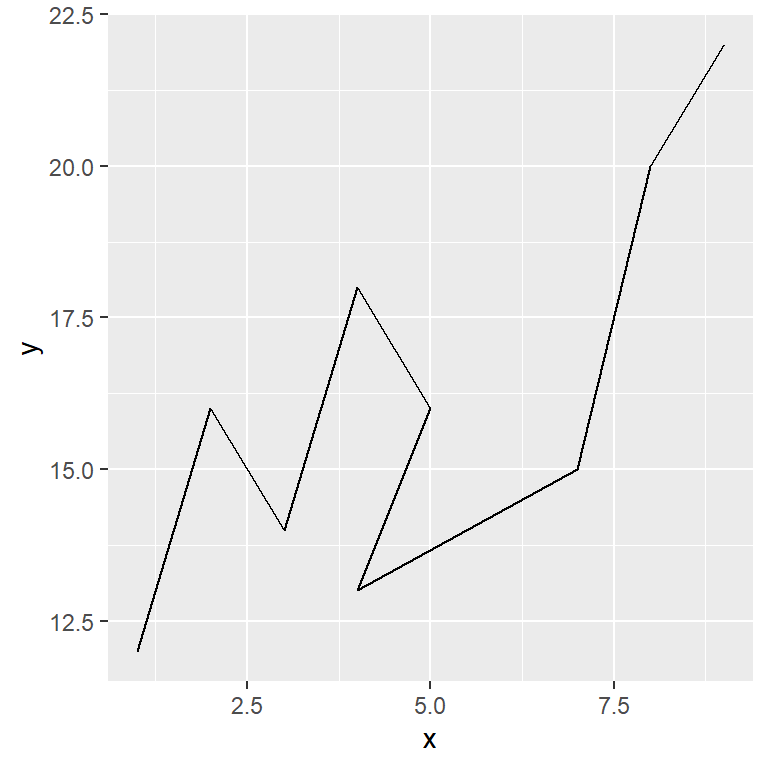Observations

You can highlight the values with `geom_point`.

``````# install.packages("ggplot2")
library(ggplot2)

# Data
x <- c(1, 2, 3, 4, 5, 4, 7, 8, 9)
y <- c(12, 16, 14, 18, 16, 13, 15, 20, 22)
df <- data.frame(x, y)

# Connected scatter plot
ggplot(df, aes(x = x, y = y)) +
geom_path() +
geom_point(size = 2)``````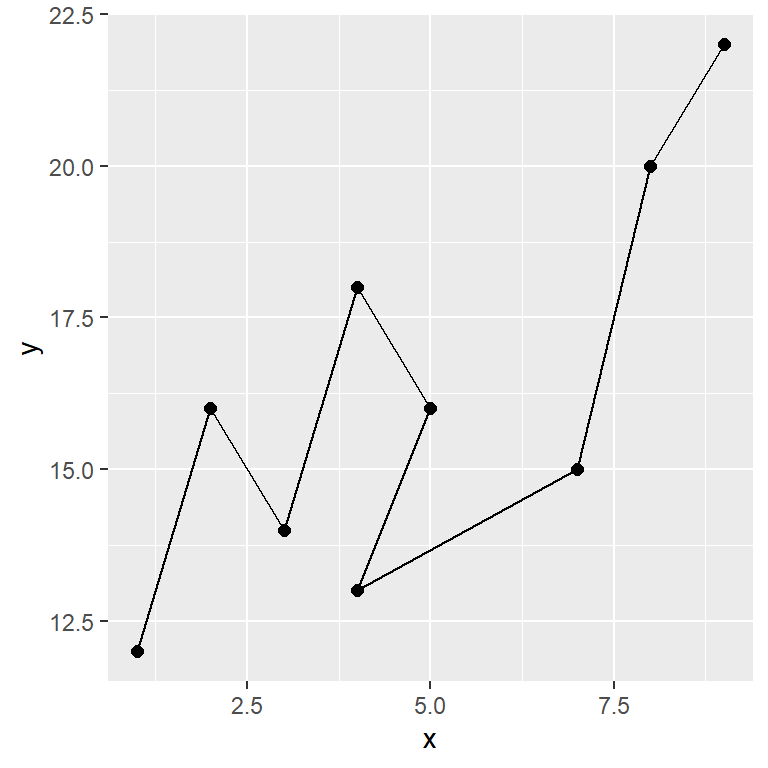Labelling points

You can label each observation making use of `geom_text`. In this example we are going to add some dates.

``````# install.packages("ggplot2")
library(ggplot2)

# Data
x <- c(1, 2, 3, 4, 5, 4, 7, 8, 9)
y <- c(12, 16, 14, 18, 16, 13, 15, 20, 22)
labels <- 2013:2021
df <- data.frame(x, y, labels = labels)

# Connected scatter plot
ggplot(df, aes(x = x, y = y)) +
geom_path(color = 4) +
geom_point(size = 2, color = 4) +
geom_text(aes(label = labels, x = x + 0.7, y = y))``````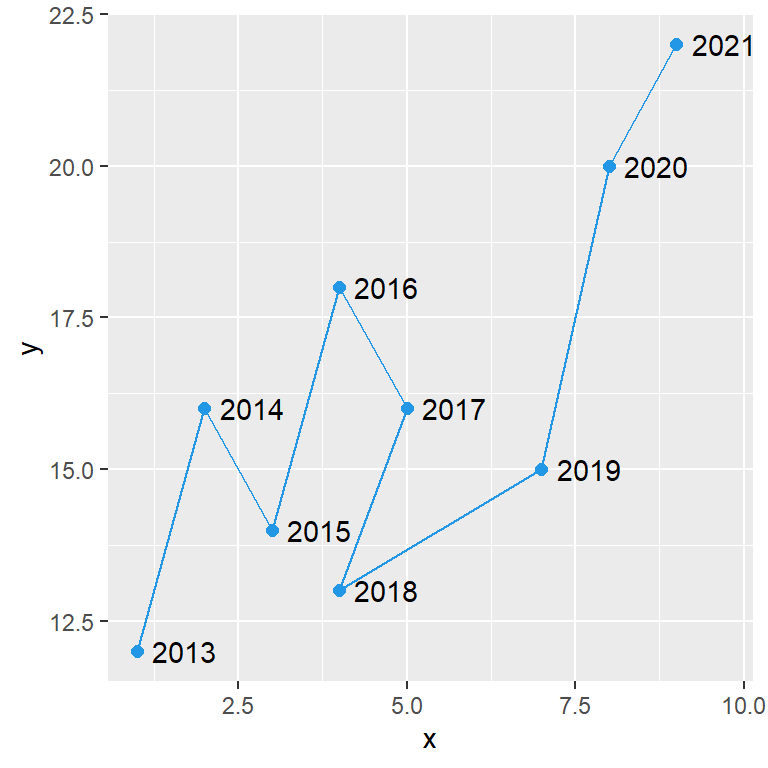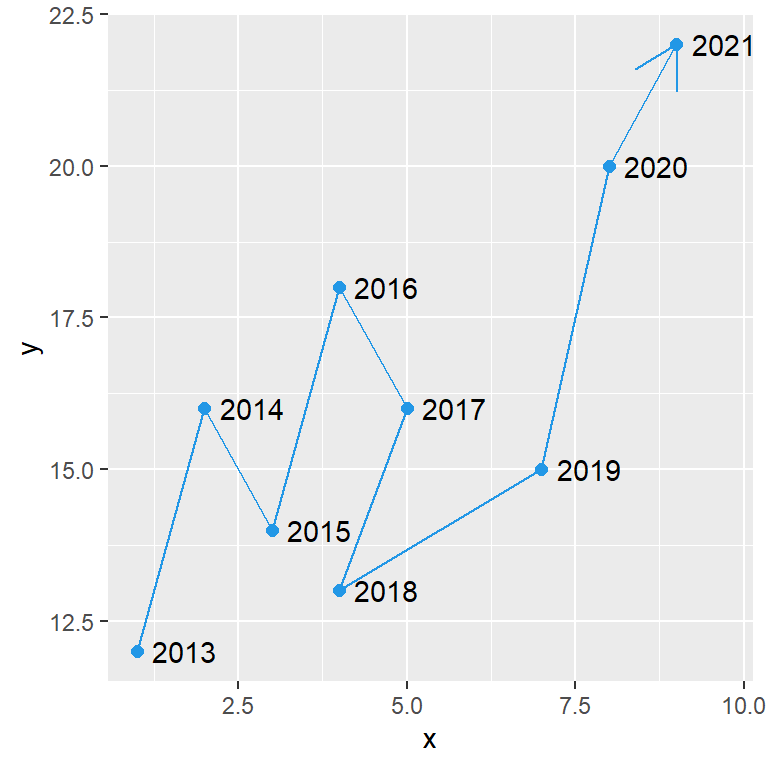You can pass the `arrow` function to the `arrow` argument of the `geom_path` function to add an arrow to the end of the path.

``````# install.packages("ggplot2")
library(ggplot2)

# Data
x <- c(1, 2, 3, 4, 5, 4, 7, 8, 9)
y <- c(12, 16, 14, 18, 16, 13, 15, 20, 22)
labels <- 2013:2021
df <- data.frame(x, y, labels = labels)

# Connected scatter plot
ggplot(df, aes(x = x, y = y)) +
geom_path(color = 4, arrow = arrow()) +
geom_point(size = 2, color = 4) +
geom_text(aes(label = labels, x = x + 0.7, y = y))``````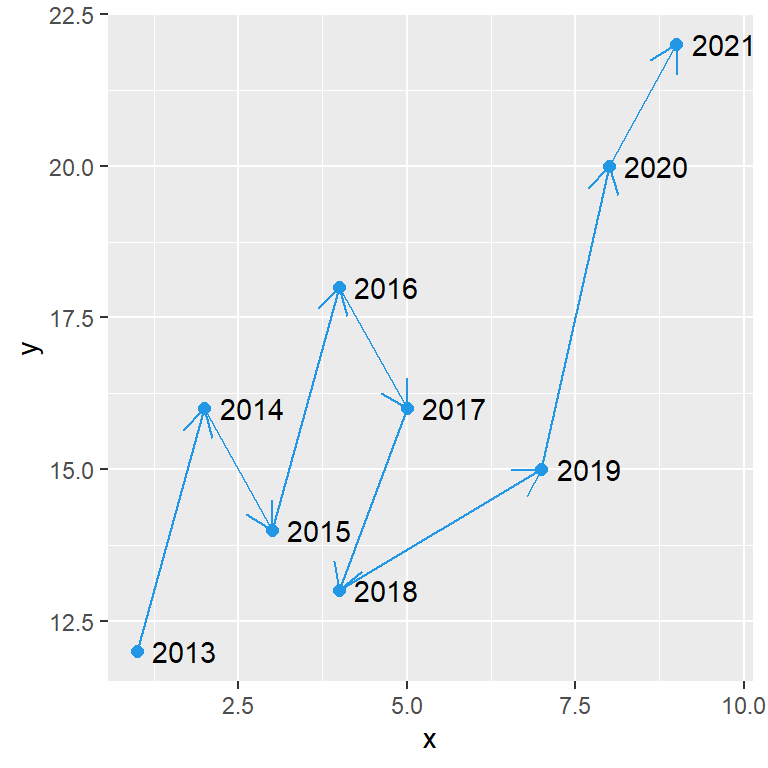However, if you use `geom_segment` as follows you can add an arrow between each pair of observations.

``````# install.packages("ggplot2")
library(ggplot2)

# Data
x <- c(1, 2, 3, 4, 5, 4, 7, 8, 9)
y <- c(12, 16, 14, 18, 16, 13, 15, 20, 22)
labels <- 2013:2021
df <- data.frame(x, y, labels = labels)

# Connected scatter plot
ggplot(df, aes(x = x, y = y)) +
geom_segment(aes(xend = c(tail(x, n = -1), NA),
yend = c(tail(y, n = -1), NA)),
arrow = arrow(length = unit(0.4, "cm")),
color = 4) +
geom_point(size = 2, color = 4) +
geom_text(aes(label = labels, x = x + 0.7, y = y))``````